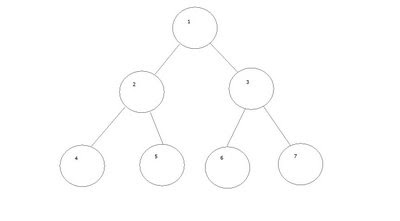Question: Write an algorithm to print a binary tree level wise and that too from leaves to root.

Example:If binary tree is as given above your answer should be: 4 5 6 7 2 3 1

`  BreadthOrderTraversal(BinaryTree binaryTree){    Queue queue;    queue.Enqueue(binaryTree.Root);    while(Queue.Size > 0)    {        Node n = GetFirstNodeInQueue();        queue.Enqueue(n.LeftChild); //Enqueue if exists        queue.Enqueue(n.RightChild); //Enqueue if exists        queue.Dequeue(); //Visit    }}`

And this will give the answer as 1 2 3 4 5 6 7, one can think of reverse of it, but it will print the nodes at a particular level in reverse order, which won't solve our purpose.

This problem can be solved by Enqueuing Right child prior to left child, i.e. :

`  BreadthOrderTraversal_1(BinaryTree binaryTree){    Queue queue;    queue.Enqueue(binaryTree.Root);    while(Queue.Size > 0)    {        Node n = GetFirstNodeInQueue();        queue.Enqueue(n.RightChild); //Enqueue if exists        queue.Enqueue(n.LeftChild); //Enqueue if exists        queue.Dequeue(); //Visit    }}`

This will give us output as 1 3 2 7 6 5 4 and reversing this will give 4 5 6 7 2 3 1.

So printing those in reverse order can be easily done by putting them in stack and print when complete tree is scanned.

`  BreadthOrderTraversalReverse(BinaryTree binaryTree){    Queue queue;    queue.Enqueue(binaryTree.Root);    while(Queue.Size > 0)    {        Node n = GetFirstNodeInQueue();        queue.Enqueue(n.RightChild); //Enqueue if exists        queue.Enqueue(n.LeftChild); //Enqueue if exists        stack.push() = queue.Dequeue(); //Visit    }    while(!isEmpty(stack))    {        process(stack.top);        stack.pop();    }}`